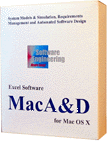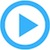# System Models & Simulation

We live in a world of systems driven by cause and affect. Those systems include financial, production, inventory, biological, chemical, thermodynamic or workflow.

Systems can be modeled as nodes representing system variables and connecting lines representing causal effects. The changing value of one variable can cause another to increase or decrease as described by equations.

Understanding how a system really works is the first step towards using, improving, automating or explaining it to others.

Causal Loop Diagrams (CLDs) are used to model dynamic systems. The simple diagram notation of nodes and lines, identifies the important variables in a system and how they interact.

Facts about the system are used to parameterize a causal loop diagram. Each node becomes a variable that identifies a quantifiable property of the system that changes over time. Each line can have equations or rules that formalize how one variable affects another.

A parameterized model has all the information needed to simulate the dynamic response of the system over a series of time increments.

The model is declarative in that the diagram, variables and equations just declare facts about the system. Contrast that with a procedural approach written in a programming language like C. With a procedural approach, algorithms would have to be developed and implemented to simulate the dynamic system response.The System Models & Simulation paper shows how to model dynamic systems, run time simulations and chart the system behavior. It has example models for population growth, bank balance, demographics, production and water management.

Use MacA&D and WinA&D to draw system models, add equations, simulate the time response and present results with tables, charts and graphs.

Learn more with the system model demonstration video.